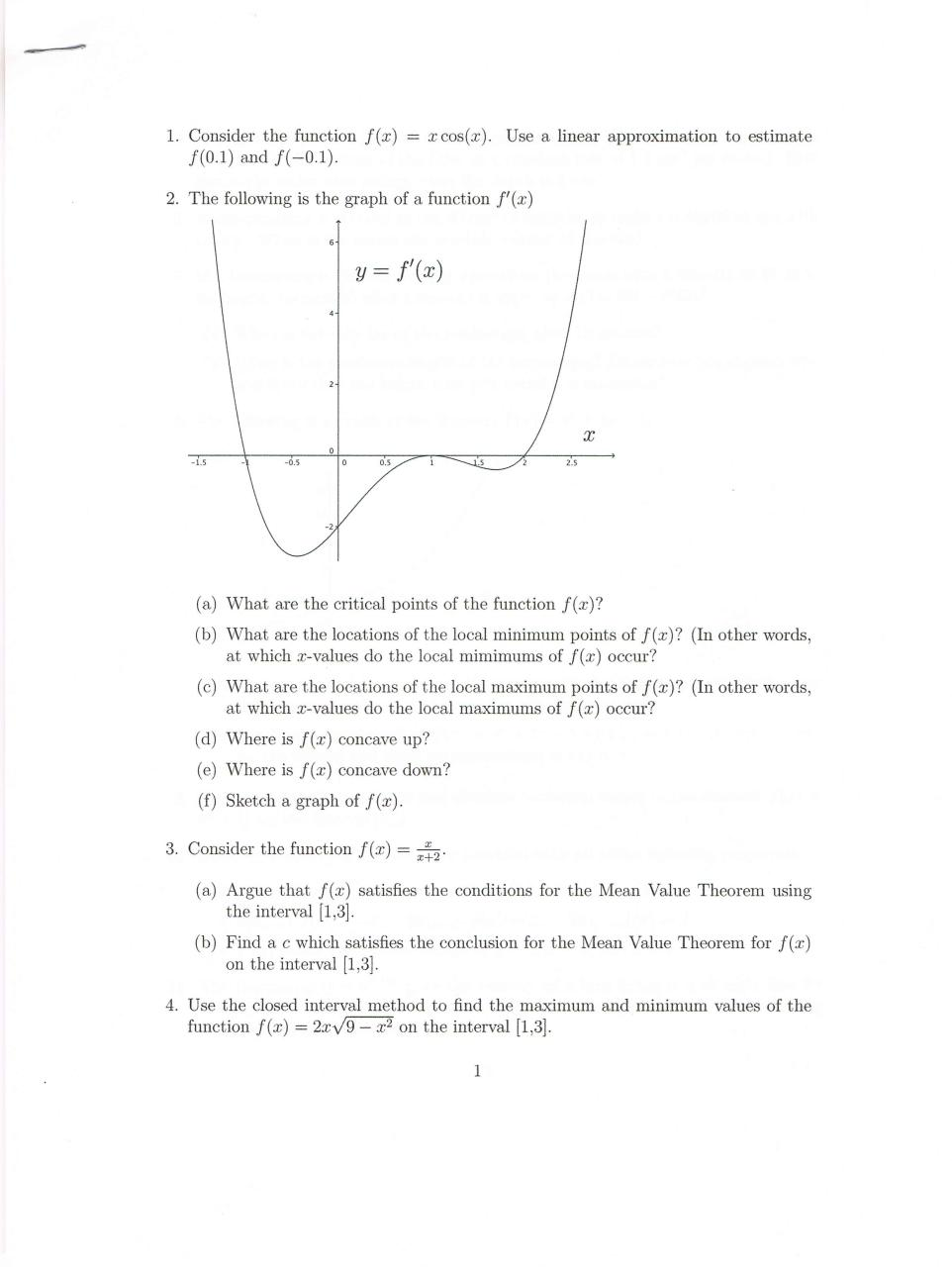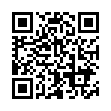# calc questions .pdf

### File information

Original filename: calc questions.pdf
Author: Jennifer Krupa

This PDF 1.3 document has been generated by PDFScanner / Mac OS X 10.8.3 Quartz PDFContext, and has been sent on pdf-archive.com on 19/05/2013 at 02:15, from IP address 50.143.x.x. The current document download page has been viewed 632 times.
File size: 313 KB (1 page).
Privacy: public file

calc questions.pdf (PDF, 313 KB)

### Document preview

1. Consider the function
f(O.1) and f(—0.1).

f

= :rcos(a3). Use a linear approximation to estimate

2. The following is the graph of a function f’(:1:)
5.

y = f'(rB)

-1'.s

critical points of the function f
(b) What are the locations of the local minimum points of f
at which a:~values do the local mimimums of f
occur?

(a) What

are the

(c) What are the locations of the local maximum points of f
at Which x-values do the local maximums of f
occur?
(cl) Where is
(e) Where is

f
f

(In other Words,
(In other words,

concave up?
concave

down?

(f) Sketch a graph of f
3. Consider the function

f

=

f satisfies the conditions for the Mean Value Theorem using
the interval [1,3].
(b) Find a c which satisfies the conclusion for the Mean Value Theorem for f
on the interval [1,3].
(a) Argue that

4. Use the closed interval method to find the maximum and minimum values of the
= 2:1:\/9 —\$2 on the interval [1,3].
function f
1Use the permanent link to the download page to share your document on Facebook, Twitter, LinkedIn, or directly with a contact by e-Mail, Messenger, Whatsapp, Line..

Use the short link to share your document on Twitter or by text message (SMS)

#### HTML Code

Copy the following HTML code to share your document on a Website or Blog

#### QR Code### Related keywords